# RD Sharma Solutions Class 11 Sets Exercise 1.2

### RD Sharma Class 11 Solutions Chapter 1 Ex 1.2 PDF Free Download

#### Exercise 1.2

Q1:Describe the following sets in Roster form:

(i){x: x is a letter before e in the english alphabet}

(ii){x $\in$ N: x2 < 25}

(iii) {x $\in$ N: x is a prime number, 10 < x < 20}

(iv) {x $\in$ N: x = 2n , n $\in[\latex] N } (v) {x \(\in$ R: x > x}

(vi) {x: x is a prime number which is a divisor of 60}

(vii){x: x is a two digit number such that the sum of its digits is 8}

(viii) The set of all letters in the word “Trigonometry”.

(ix)The set of all letters in the word “Better”.

Solution:

(i)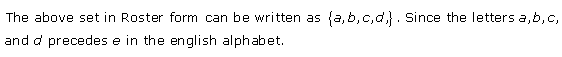(ii)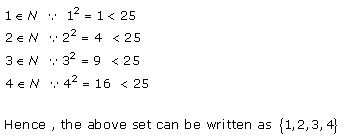(iii)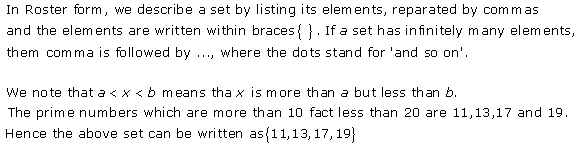(iv)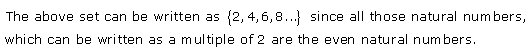(v)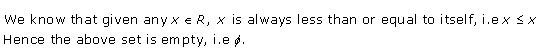(vi)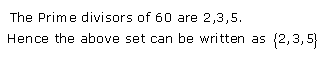(vii)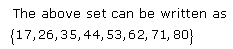(viii)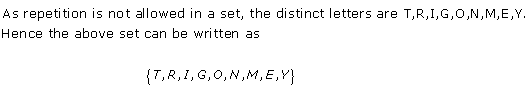(ix)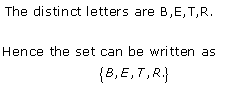Q2: Describe the following sets in set-builder form:

(i) A = {1,2,3,4,5,6}

Solution: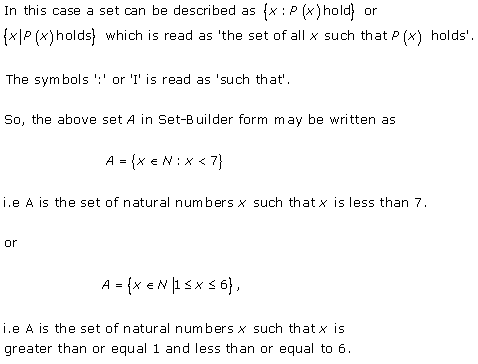(ii) B = {1,1/2,1/3,1/4,¼,……}

Solution: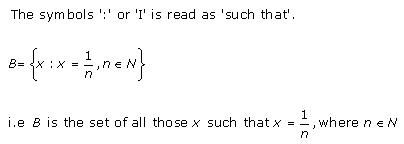(iii) C = {0,3,6,9,12,……}

Solution: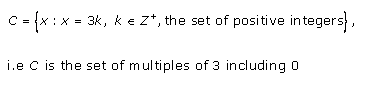(iv) D = {10,11,12,13,14,15}

Solution: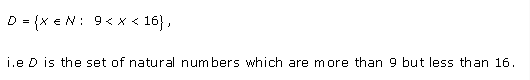(v) E = {0}

Solution: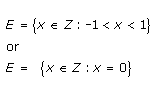(vi) {1,4,9,16,…..,100}

Solution: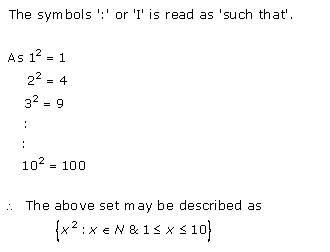(vii) {2,4,6,8,…}

Solution:

{x : x = 2n, n $\in$ N}

(viii) {5, 25, 125, 625}

Solution:

{5n : n $\in$ N ,1 ≤ n ≤ 4}